# Snarks

Relevant articles: , , .

# Snarks

Results have been obtained for a number snarks. The spectrum problem is completely solved for the Petersen graph, the Tietze graph, the two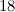$18$-vertex Blanusa snarks, the six snarks on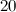$20$ vertices, the twenty snarks on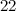$22$-vertices, and Goldberg's snark 3. Partial results have also been obtained on the two Celmin's-Swart snarks, the two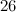$26$-vertex Blanusa snarks, the flower snark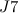$J7$, the double star snark, the two$34$-vertex Blanusa snarks, Zamfirescu's graph, Goldberg's snark 5, the Szekeres snark, and the Watkins snark.

## Spectrum Results

Table 1 summarises the known results on the spectrum problem for various snarks. The reference for each of these results is , except that the spectrum problem for the Petersen graph was solved in .

Designs covered by Wilson’s Theorem  are ignored in the listed possible exceptions in Table 1.

Table 1
 Graph Spectrum Possible exceptions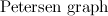${\rm Petersen\ graph}$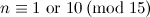$n \equiv 1 {\rm \ or \ } 10 \,({\rm mod \ }15)$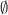$\emptyset$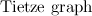${\rm Tietze\ graph}$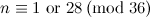$n \equiv 1 {\rm \ or \ } 28 \,({\rm mod \ }36)$$\emptyset$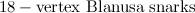${\rm 18-vertex\ Blanusa\ snarks}$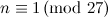$n \equiv 1 \,({\rm mod \ }27)$$\emptyset$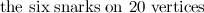${\rm the\ six\ snarks\ on\ 20\ vertices}$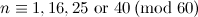$n \equiv 1,16,25 {\rm \ or \ } 40 \,({\rm mod \ }60)$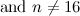${\rm and \ } n\neq 16$$\emptyset$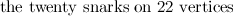${\rm the\ twenty\ snarks\ on\ 22\ vertices}$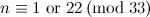$n \equiv 1 {\rm \ or \ } 22 \,({\rm mod \ }33)$$\emptyset$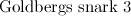${\rm Goldbergs\ snark\ 3}$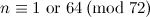$n \equiv 1 {\rm \ or \ } 64 \,({\rm mod \ }72)$$\emptyset$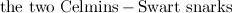${\rm the\ two\ Celmins-Swart\ snarks}$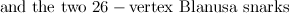${\rm and\ the\ two\ 26-vertex\ Blanusa\ snarks}$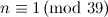$n \equiv 1 \,({\rm mod \ }39)$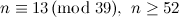$n\equiv 13 \,({\rm mod \ }39),\ n\geq 52$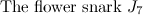${\rm The\ flower\ snark\ } J_7$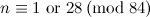$n \equiv 1 {\rm \ or \ } 28 \,({\rm mod \ }84)$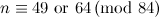$n \equiv 49 {\rm \ or \ } 64 \,({\rm mod \ }84)$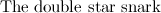${\rm The\ double\ star\ snark}$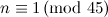$n \equiv 1 \,({\rm mod \ }45)$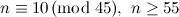$n \equiv 10 \,({\rm mod \ }45),\ n\geq 55$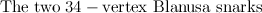${\rm The\ two\ 34-vertex\ Blanusa\ snarks}$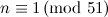$n \equiv 1 \,({\rm mod \ }51)$$n \equiv 34 \,({\rm mod \ }51)$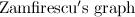${\rm Zamfirescu's\ graph}$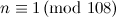$n \equiv 1 \,({\rm mod \ }108)$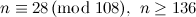$n \equiv 28 \,({\rm mod \ }108),\ n\geq 136$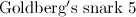${\rm Goldberg's\ snark\ 5}$$n \equiv 1 {\rm \ or \ } 40 \,({\rm mod \ }120)$$n \equiv 16 {\rm \ or \ } 25 \,({\rm mod \ }120),\ n\geq 136$${\rm The\ Szekeres\ snark}$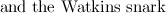${\rm and\ the\ Watkins\ snark}$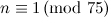$n \equiv 1 \,({\rm mod \ }75)$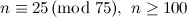$n \equiv 25 \,({\rm mod \ }75),\ n\geq 100$

## References

1. 1.0 1.1 Adams, P. and Bryant, D. E. The spectrum problem for the Petersen graph, J. Graph Theory, 22, 175–180 (1996).
2. 2.0 2.1 Forbes, A. D. Snark Designs, Preprint,
3. 3.0 3.1 Wilson, R. M. Decompositions of complete graphs into subgraphs isomorphic to a given graph, Proceedings of the Fifth British Combinatorial Conference (Univ. Aberdeen, Aberdeen, 1975), Congressus Numerantium, No. XV, Utilitas Math., Winnipeg, Man. 647–659 (1976).